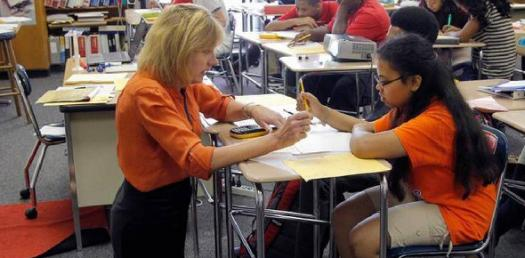# Test Quiz For 5th Grade Math

15 Questions | Total Attempts: 150SettingsDo you know all the facts about 5th grade math? Take this quiz and prove yourself!

• 1.
Which of the following is equivalent to 4500 pounds?
• A.

2 Tons 1000 Pounds

• B.

4.5 Tons

• C.

4 Tons 500 Pounds

• D.

2 Tons 500 Pounds

• 2.
What is the area of a triangle that has a height of 9 feet and a base of 12 feet?
• A.

108 Square Feet

• B.

54 Square Feet

• C.

216 Square Feet

• D.

21 Square Feet

• 3.
What is 2/3 times 4?
• A.

3-Aug

• B.

12-Aug

• C.

3-Feb

• D.

12-Feb

• 4.
What is the cost of a \$90 pair of shoes after a 10% discount?
• A.

89

• B.

85

• C.

80

• D.

81

• 5.
If a discounted CD is priced \$7.20 with an additional sale of 40% off, what is the new price?
• A.

4.32

• B.

8.25

• C.

4.15

• D.

5.53

• 6.
How many degrees are in the angle that the hands of a clock make at three o'clock?
• A.

75

• B.

100

• C.

180

• D.

90

• 7.
What is 10% of 2380?
• A.

2.38

• B.

23.8

• C.

238

• D.

23800

• 8.
In a grid of 100 squares, how many squares should be shaded in for 15% of the grid to be shaded?
• A.

30

• B.

15

• C.

5

• D.

50

• 9.
If you invest \$5,000 at a rate of 6%, how much interest will you have earned after one year?
• A.

500

• B.

100

• C.

300

• D.

700

• 10.
How many words are there in a book with 200 pages and an average of 120 words per page?
• A.

24,400

• B.

20,400

• C.

24,000

• D.

20,040

• 11.
John bought a plane ticket for \$150. How much does John pay overall if tax rate is 9%?
• A.

170

• B.

163.5

• C.

160.25

• D.

165

• 12.
A boy is 4 times the age of a girl. In 4 years, he will be twice as old as her. How old is the boy?
• A.

10

• B.

4

• C.

8

• D.

12

• 13.
If a computer costs \$1800 and is discounted by 5 per cent, what is the final price?
• A.

1780

• B.

1600

• C.

1710

• D.

1000

• 14.
If Jen has four sweaters and three skirts, and they all go together, how many outfits can she make?
• A.

Seven

• B.

12

• C.

81

• D.

256

• 15.
If a basketball player hits 50 out of 60 free throws, what percent of her free throws does she make?
• A.

75%

• B.

60%

• C.

83%

• D.

50%

Related TopicsBack to top